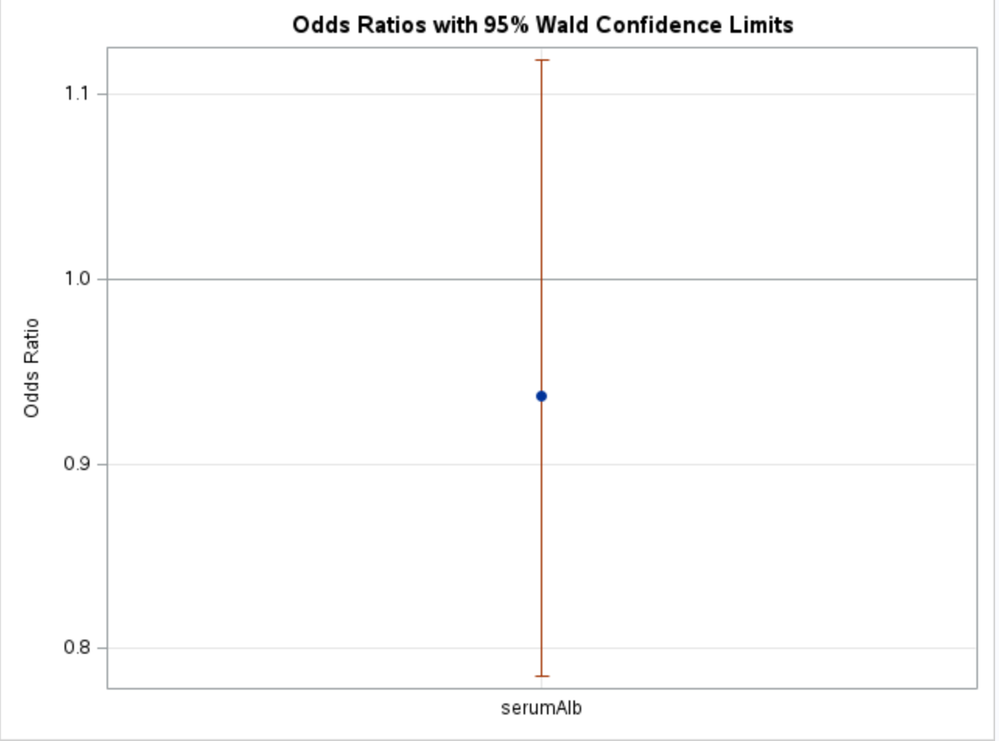## spline for odd ratios after logistic regression

Hi, everyone

I spent a lot of time yesterday searching how to make spline for odd ratios after a logistic regression. My idea is simple the coefficient of a continuous variable is derived from the logistic regression, and I can use it and its CI to construct the spline for ORs.

But I did not find any direct SAS procedures or statements to do so. Would you guide me the direction? Thank you.

1 ACCEPTED SOLUTION

Accepted Solutions

## Re: spline for odd ratios after logistic regression

The most likely reason is that you specified serumALB in your MODEL statement as a predictor rather than the spline effect, splALB. Effectively, the spline was not even used in the model.
6 REPLIES 6Ksharp
Super User

## Re: spline for odd ratios after logistic regression

Better post it at Statistic Forum:
https://communities.sas.com/t5/Statistical-Procedures/bd-p/statistical_procedures
@StatDave maybe could give you a hand.

## Re: spline for odd ratios after logistic regression

What you are trying to do is not entirely clear, but this note might address it.

## Re: spline for odd ratios after logistic regression

Hello, Dave.

Wow! That's a great reference. Thanks. I copied and edited the code to suit my project. But, so far, I have never get the Odd Ratio plot as shown in the first example. My code only generated one column for the variable of serumAlb. I am just not able to make it. Your investigation would be of great important. Thanks.

My code:

``````%macro Logistic2;
proc logistic data= &raw_data_name plots = oddsratio(type=vertical);
class &class_var;
effect SplALB=spline(serumAlb/naturalcubic);
model &censor(event = '1') = &indep_list;
effectplot fit(x=serumAlb);
oddsratio serumAlb / at(serumAlb=34.8125 36.0425 37.35 39.0 40.92);
store kmod;
run;
%mend;
%Logistic2``````## Re: spline for odd ratios after logistic regression

You got to work incrementally and get something basic to work before tailoring it to your cause. Try copying the syntax in the note exactly except for your variable and dataset. Will it work? If so, you can make little incremental changes, all the time saving the previous working codes for possible step back. For example, the values in the note had “to” syntax. Also, do not use macro language at early development stage. Everything should be unoriginal, clear and transparent.

## Re: spline for odd ratios after logistic regression

The most likely reason is that you specified serumALB in your MODEL statement as a predictor rather than the spline effect, splALB. Effectively, the spline was not even used in the model.

## Re: spline for odd ratios after logistic regression

Exactly! This is the issue. I specified serumALB instead of SplALB in the MODEL statement. After correcting this error, everything followed as expected. Thank you.
Discussion stats
• 6 replies
• 231 views
• 3 likes
• 4 in conversation